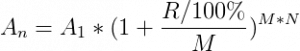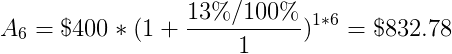# Compound Interest Calculation

## Compound interest calculation formula:A1 – initial amount
An – amount after n years (or days/weeks/months)
R – nominal annual interest rate
M – number of compounding periods in one year
N – number of years (or days/weeks/months)

Example #1

Initial amount (A1) = \$400
Number of years (N) = 6
Nominal annual interest rate (R), compounded yearly = 13%

So,

Number of compounding periods in one year (M) = 1
Amount after 6 years:Interest amount = \$832.78 – \$400 = \$432.78
Total yield = (\$832.78 – \$400) / \$400 x 100% = 108.2%

Example #2

Initial amount (A1) = \$400
Number of years (N) = 5
Nominal annual interest rate (R), compounded monthly = 13%

So,

Number of compounding periods in one year (M) = 12
Amount after 5 years:Interest amount = \$763.54 – \$400 = \$363.54
Total yield = (\$763.54 – \$400) / \$400 x 100% = 90.9%

Example #3

Initial amount (A1) = \$400
Number of months (N) = 5
Nominal annual interest rate (R), compounded monthly = 13%

So,

Number of compounding periods in one year (M) = 12
Amount after 5 months:Interest amount = \$422.14 – \$400 = \$22.14
Total yield = (\$422.14 – \$400) / \$400 x 100% = 5.54%